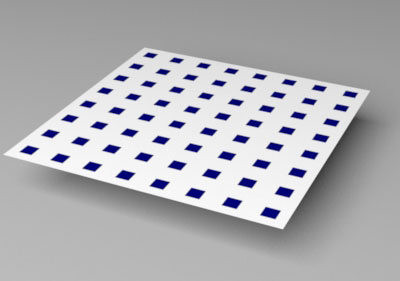### OSLRepeating Patterns

#### Introduction

For the purpose of illustrating how repeating patterns are generated the code shown in listing 1 will be modified so that it produces not just one square, figure 1, but many squares, figure 2.Figure 1Figure 2

Listing 1

 ```// Returns a value in the range 0 to 1. // 0 indicates the x,y point is outside the square, and // 1 indicates the x,y point is fully within the squere. float pntInSquare(float x, float y, float x_center, float y_center, float x_width, float y_width, float blur) { float minx = x_center - x_width/2; float maxx = x_center + x_width/2; float miny = y_center - y_width/2; float maxy = y_center + y_width/2; return smoothstep(minx, minx + blur, x) * (1 - smoothstep(maxx, maxx + blur, x)) * smoothstep(miny, miny + blur, y) * (1 - smoothstep(maxy, maxy + blur, y)); } //---------------------------------------------------- shader square(float s_center = 0.5, float t_center = 0.5, float s_width = 0.4, float t_width = 0.4, float blur = 0.01, color bakcolor = 1, color patcolor = 0, float s = 0 [[int lockgeom = 0]], float t = 0 [[int lockgeom = 0]], output color resultRGB = 0) { float blend = pntInSquare(s, t, s_center, t_center, s_width, t_width, blur); resultRGB = mix(bakcolor, patcolor, blend); } ```

##### Repetitions

Repetitions are based on the use of the `mod`() function. The function divides one number by another and returns the remainder. For example,

`    mod(3, 2);`

returns the value 0.5 - the remainder of 3 divided by 2. The function can be used to remap values in the range 0 to 1 to a sequence of repeating sub-ranges. In each sub-range the maximum value becomes infinitly close to 1 but never actually reaches that value.To generate 8 repeating patterns, say in the '`s`' direction, the following expression could be applied.

`    float ss = mod(s * number_of_repeats, 1);`

Using 1 as the divider ensures we obtain the correct remainder. The effect of using mod() in both '`s`' and '`t`', with a repetition of 8, is shown in figure 2..

Modifying the "square" shader, listing 1, so that it can render repeating squares is just a matter of passing remapped versions of 's' and 't' to the pntInSquare() function. For example,

```    float ss = mod(s * number_of_repeats, 1);
float tt = mod(t * number_of_repeats, 1);
float blend = pntInSquare(ss, tt, s_center, t_center, s_width, t_width, blur);```

The full code of a "squares" shader is shown in listing 2.

Listing 2

 ```shader squares(float s_center = 0.5, float t_center = 0.5, float s_width = 0.4, float t_width = 0.4, float blur = 0.01, color bakcolor = 1, color patcolor = color(0,0,0.2), float s_repeats = 8, float t_repeats = 8, float s = 0 [[int lockgeom = 0]], float t = 0 [[int lockgeom = 0]], output color resultRGB = 0) { float ss = mod(s * s_repeats, 1); float tt = mod(t * t_repeats, 1); float blend = pntInSquare(ss, tt, s_center, t_center, s_width, t_width, blur); resultRGB = mix(bakcolor, patcolor, blend); }```